GFG App
Open AppBrowser
Continue

# How to Plot Bivariate Data in Excel?

Bivariate data is the most used type of data representation used for the plotting of scatter plots. The data depends on two variables, and it is analyzed using different machine learning algorithms, using different charts, etc. Linear regression is one of the most used machine learning algorithms for bivariate data. In this article, we will learn how to deal with bivariate data, and analyze it using scatter plots.

## Bivariate Data

Bivariate data is data which have two variable dependencies. The data can be either Quantitative or Qualitative. The value of one variable changes accordingly with the value of the second variable. The Quantitative bivariate data can be represented in the form of a scatter plot, and Qualitative variable data can be represented in the form of a frequency distribution table. A correlation can exist in bivariate data, the correlation value ranges from -1 and 1.

## Scatter Plots

Scatter plots are graphs that represent the relation between two variables. It is simply said as plotting points, on the X and Y axis. They are very useful for data analysis or regression analysis. The independent attributes are plotted on X-axis, while dependent attributes are plotted on Y-axis. For example, you are given a scatter plot of Study Hours and Marks(100).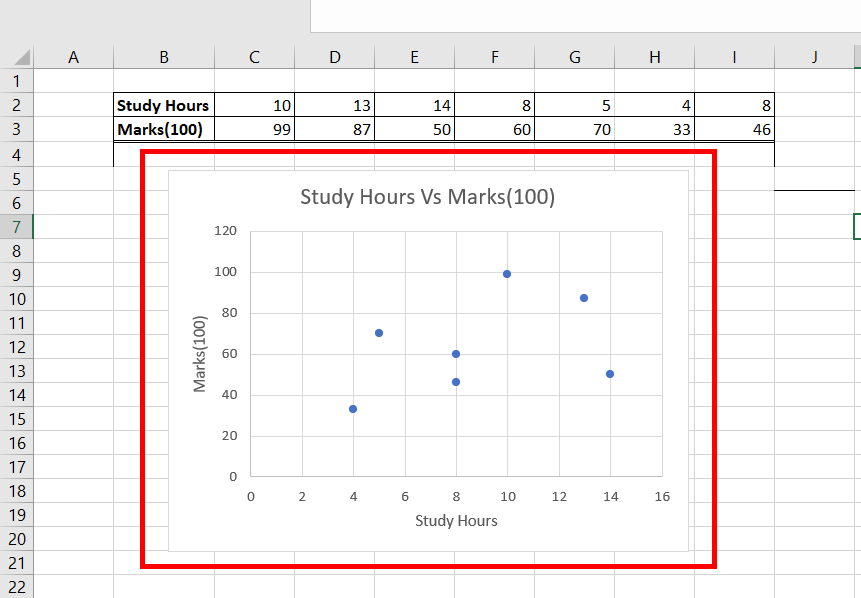## Linear Regression

Linear Regression is a machine learning model, which is used to predict the future values of a dependent variable, with respect to the best fit line. The best fit line is the line that passes through the data points, such that they have the minimum distance error sum from all the points. This is also called a trend line. Excel one-click functionality, to display the equation of the trend line, which is achieved from the machine learning linear regression algorithm.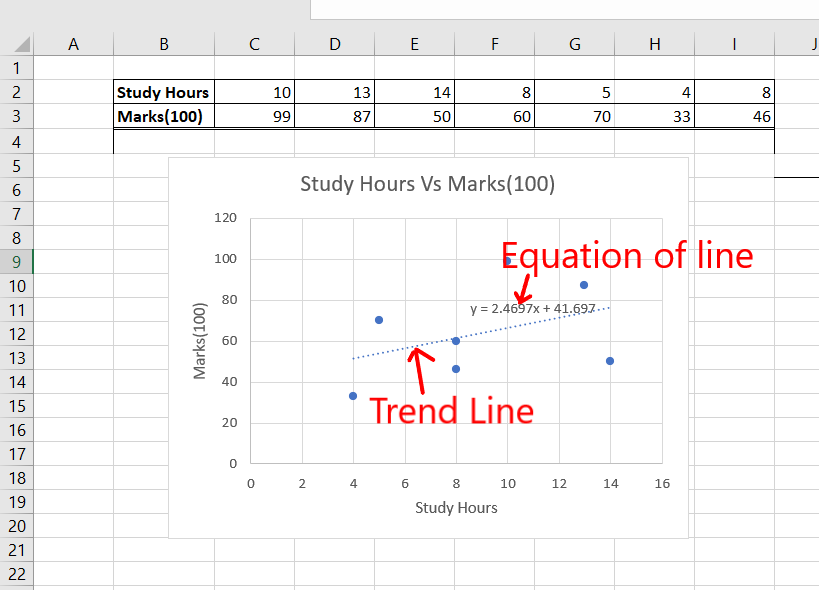## Plotting Bivariate Data in Scatter Plot

Now, we will learn how to create a scatter plot, and add a trend line, which will help us analyze the bivariate data in a better way. For example, Arushi is the class teacher of the tenth (X)th class, she collected data from her students, how many hours they use to study in a day, and added the marks achieved corresponding to that student, her task is to analyze this bivariate data in excel, by plotting it in a scatter plot.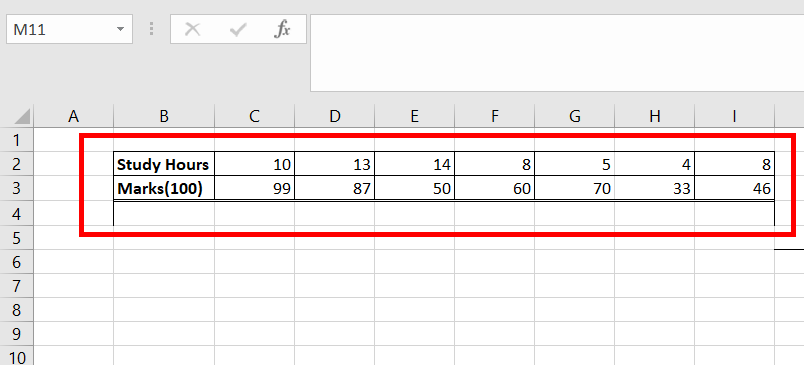Following are the steps:

Step 1: Select the data, and go to the Insert Tab, to add a scatter chart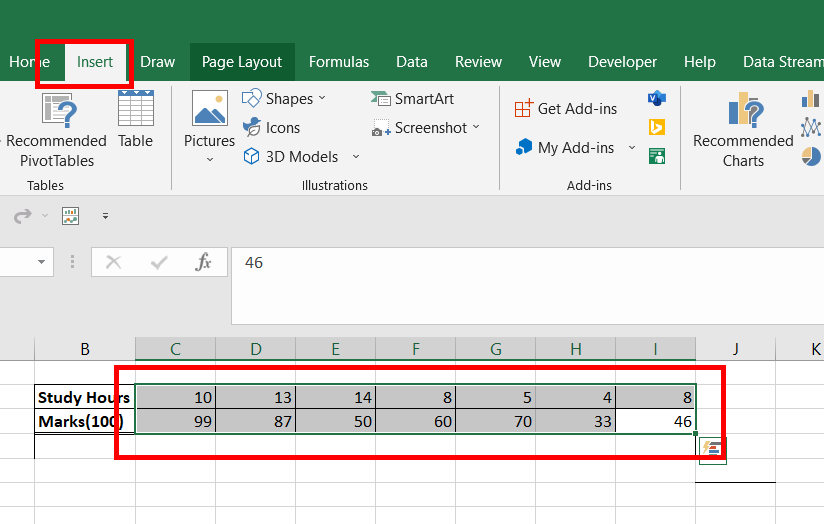Step 2: Under the Charts section, select the Scatter chart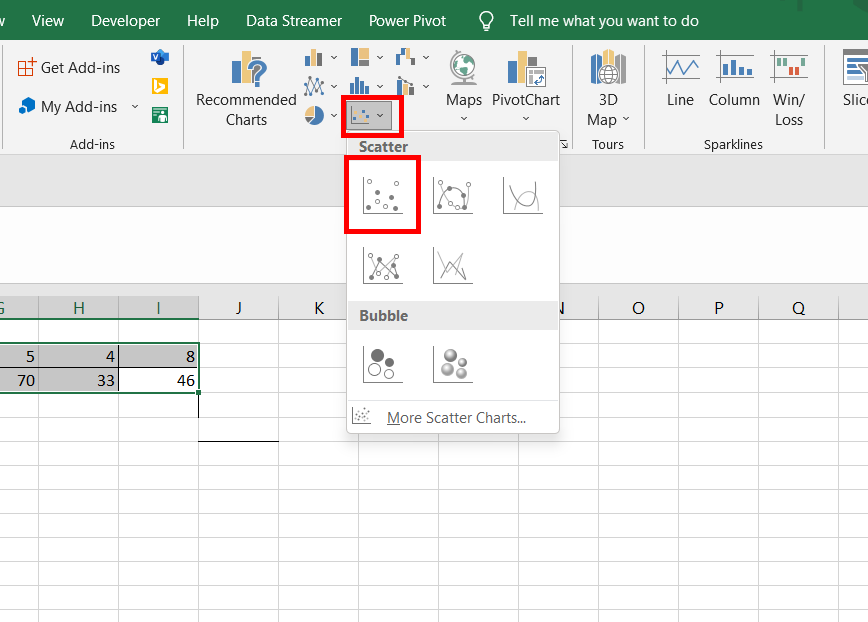Step 3: A chart is created, between Study Hours (X-axis) and Marks Scored (Y-axis)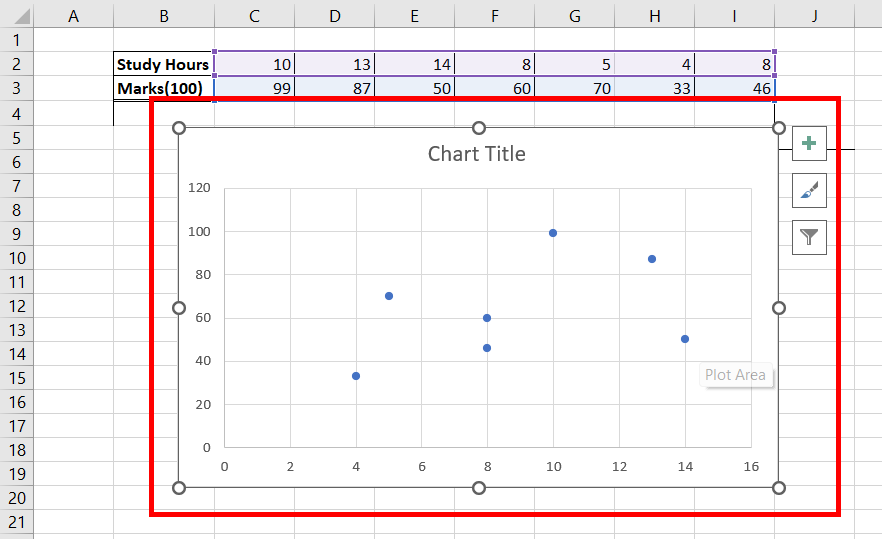Step 4: Add a custom title to the prepared chart i.e. Study Hours Vs Marks(100)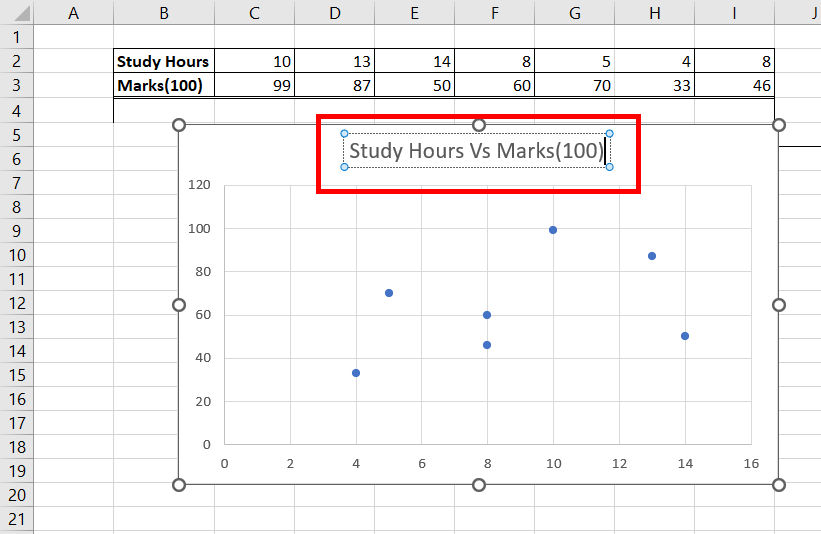Step 5: Select the chart, click on the plus(+) button, and check the box Axis Titles. This adds the name of the X-Axis and Y-Axis.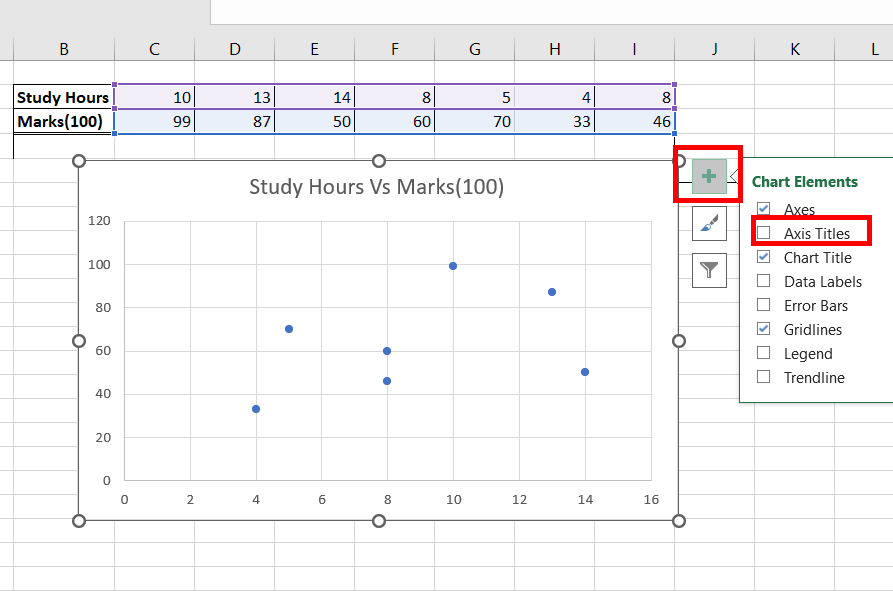Step 6: The Axis titles, box is added, with the default name as Axis Title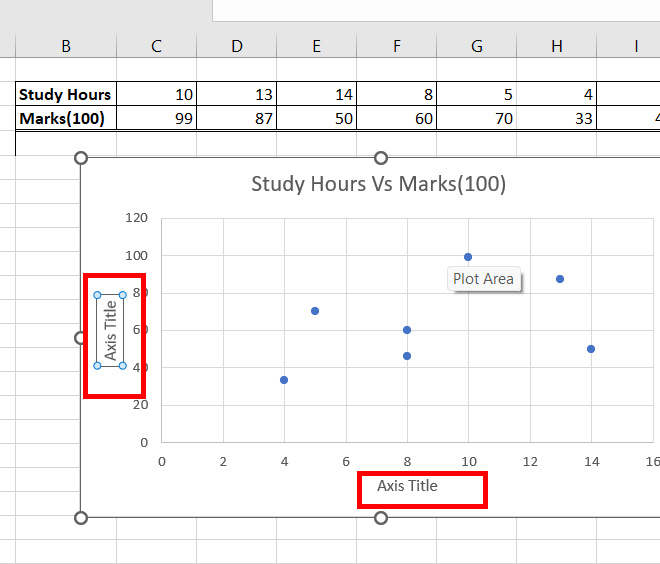Step 7: On the X and Y axis, select the Axis Title, and change the name to Study Hours and Marks(100) respectively.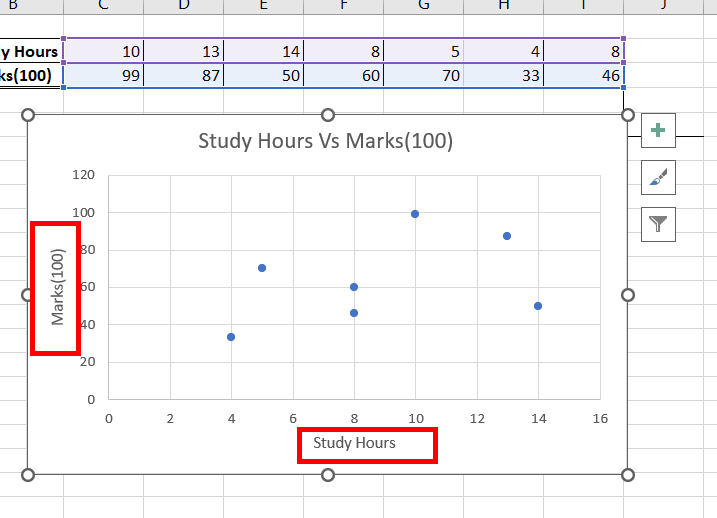Step 8: Select the X-Axis chart area, and right-click on it. A drop-down appears, select the format axis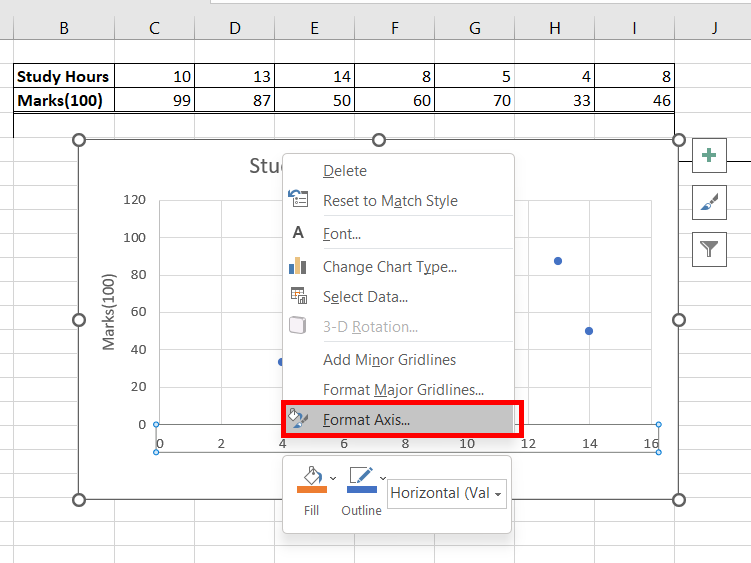Step 9: A Format Axis, dialogue box appears, at the right-most side of your screen. Under the Axis Options, the default value of minimum is 0. We can see our data starts after value 4. To have better visualization, we could change the minimum value from 0 to 3. This totally depends on the user, one can provide any value as the minimum value.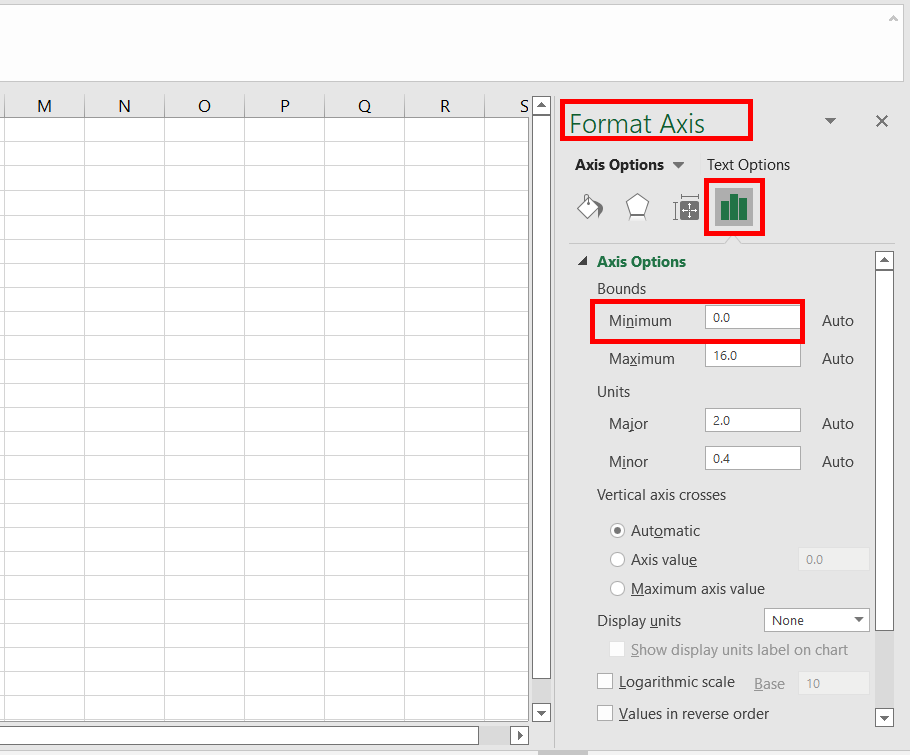Step 10: Change the minimum value from 0 to 3. Press EnterStep 11: Now, you can observe, our X-axis starts from value 3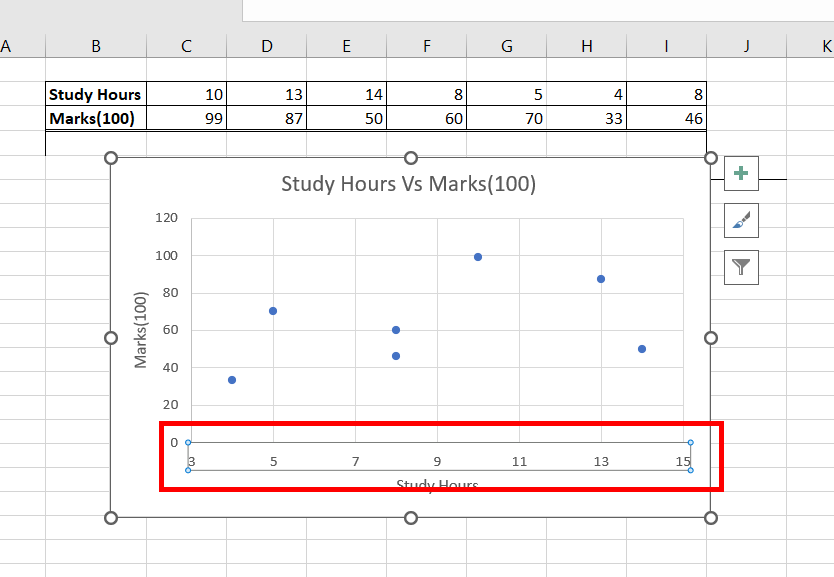Step 12: Repeat the same for Y-axis. Select the Y-axis chart area, and right-click on it. A drop-down appears, select the format axis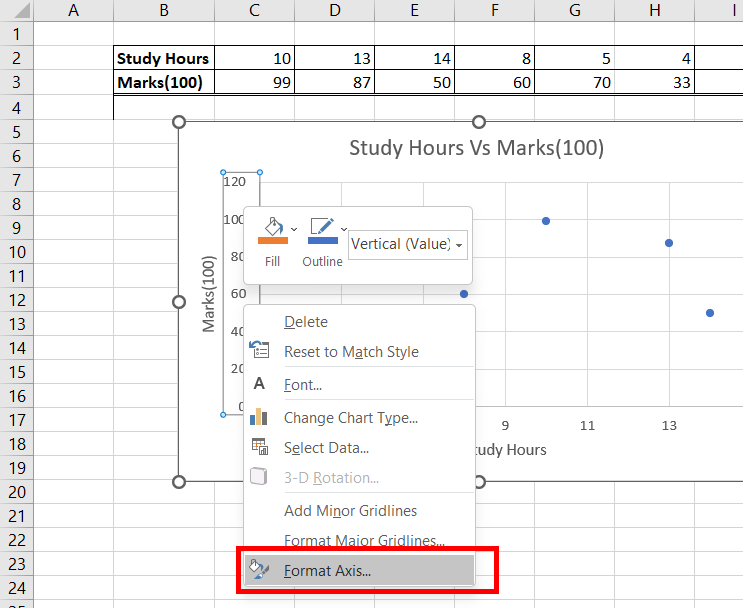Step 13: A Format Axis, a dialogue box appears, at the right-most side of your screen. Under the Axis Options, the default value of minimum is 0. We can see our data starts after value 40. To have better visualization, we could change the minimum value from 0 to 30. This totally depends on the user, one can provide any value as the minimum value. Press Enter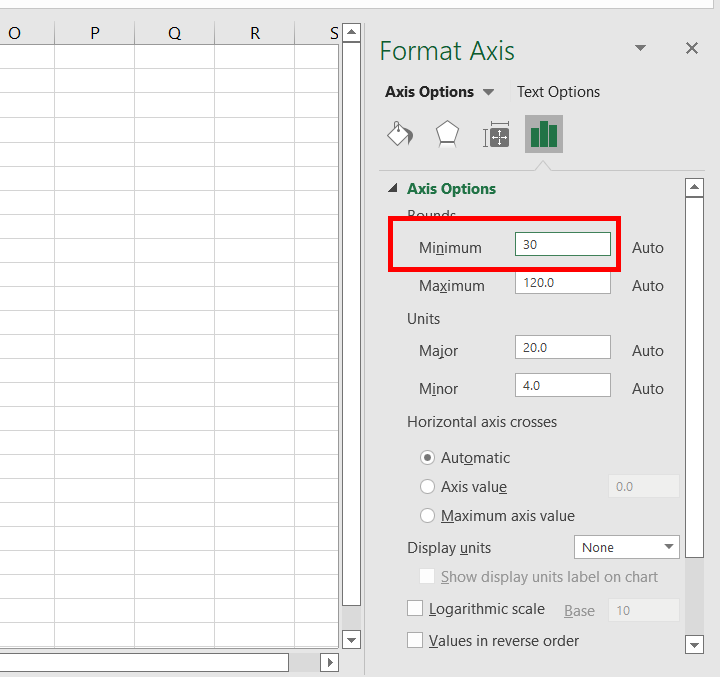Step 14: Now, you observe, our Y-axis starts from value 30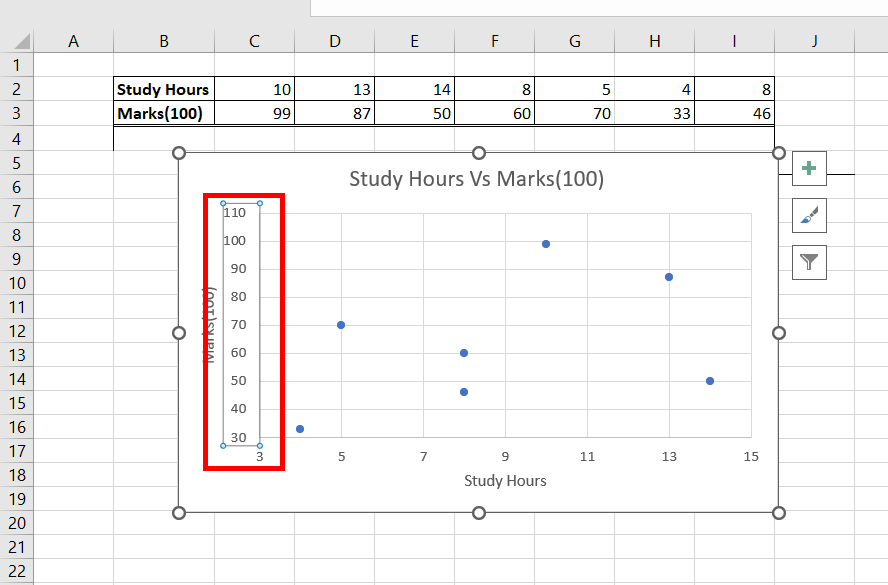Step 15: Now, the only work left is to add a trend line, and an equation of the best fit line, which is part of our linear regression. Select the data points, and right-click on them. Select Add Trendline. This adds the trend line to your chart.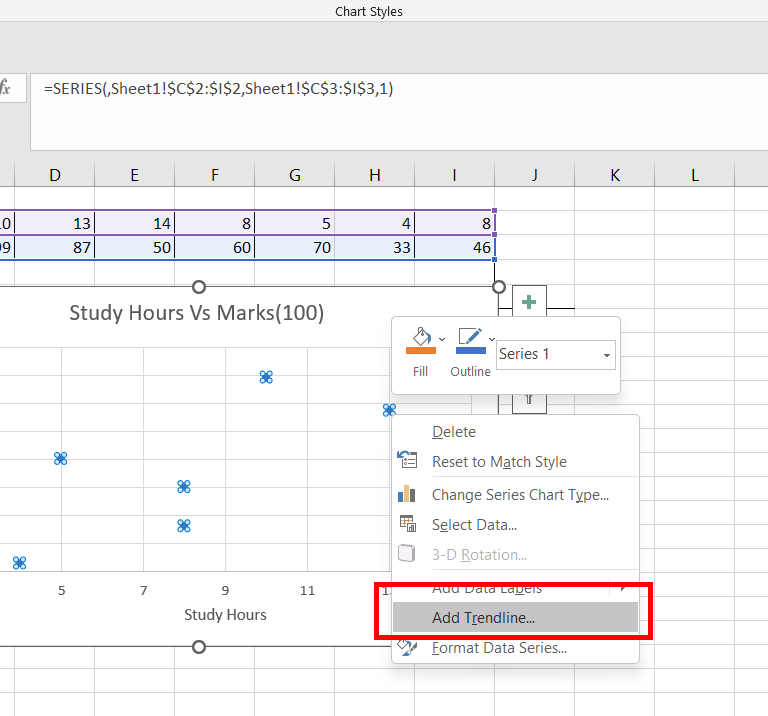Step 16: Also, a Format Trendline dialogue box appears, at the right-most side of your screen. Under the Trendline Options, check the box, Display equation on the chart.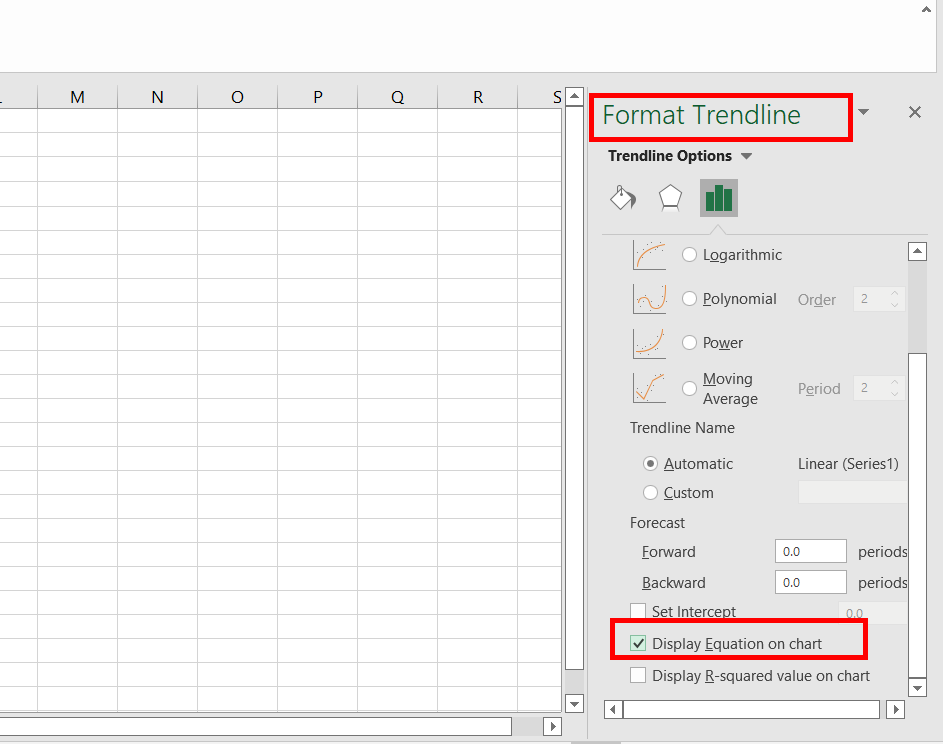Step 17: A Trend line and its equation is added to your chart.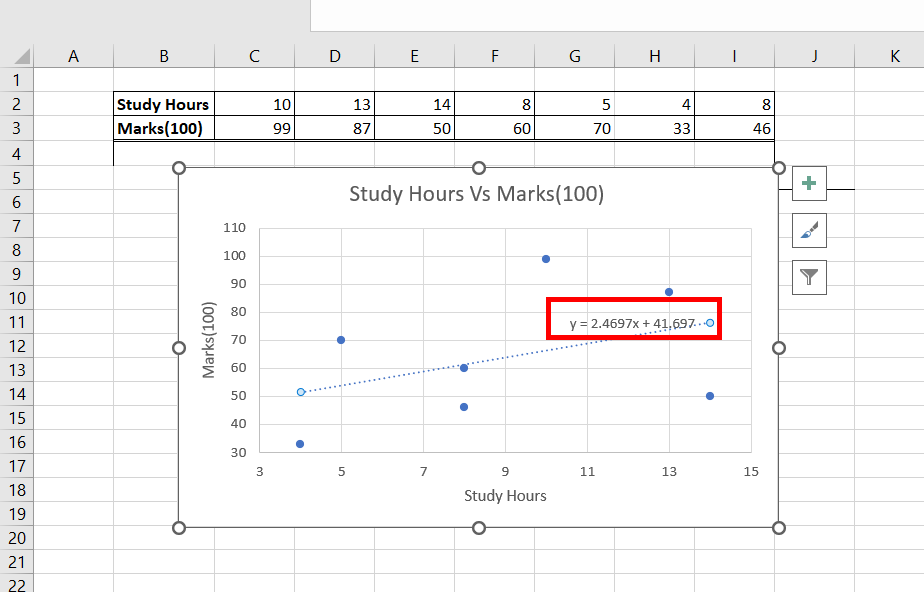Step 18: Our Bivariate data is plotted, efficiently for analytical purposes. The only work left is to add the correlation value between the two variables. Use =CORREL(C2:I2, C3:I3) function, to add a correlation between the variables. Press Enter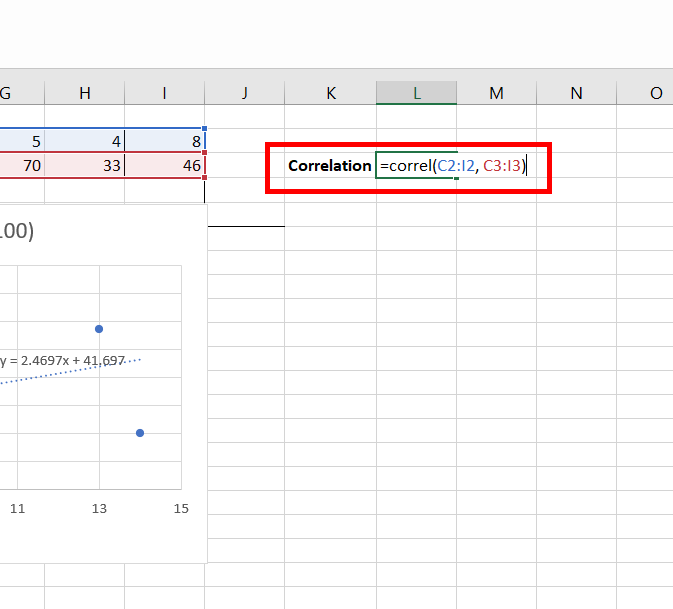Step 19: The correlation value is 0.396 i.e. positive, which signifies that as the study hours increase, the marks of students also increase.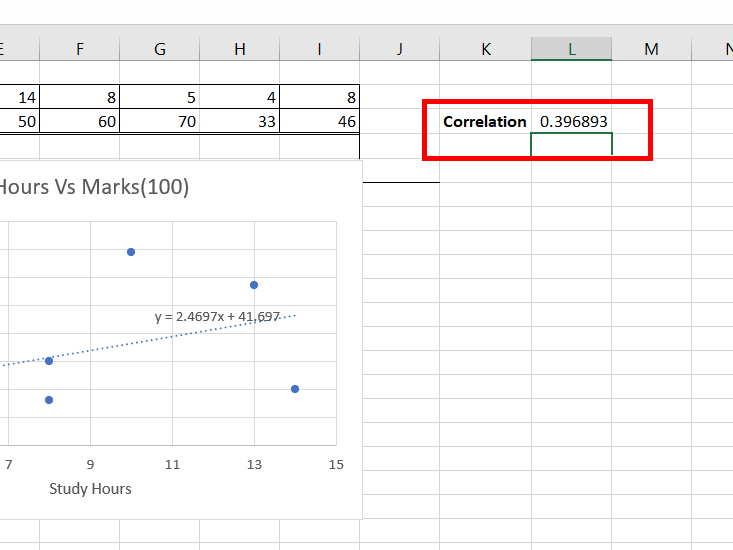My Personal Notes arrow_drop_up